# 创建包含双 y 轴的图。

### 绘制数据对左侧 y 轴的图。

```x = linspace(0,25); y = sin(x/2); yyaxis left plot(x,y);```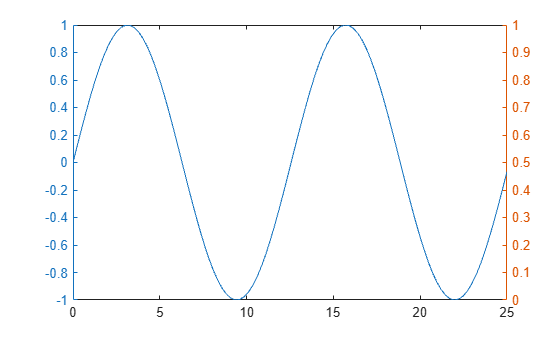### 绘制数据对右侧 y 轴的图。

```r = x.^2/2; yyaxis right plot(x,r);```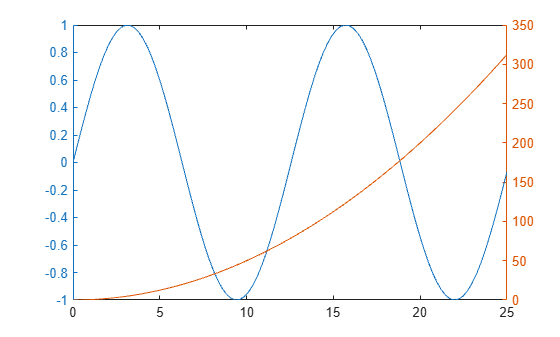### 添加标题和轴标签

```yyaxis left title('Plots with Different y-Scales') xlabel('Values from 0 to 25') ylabel('Left Side') yyaxis right ylabel('Right Side')```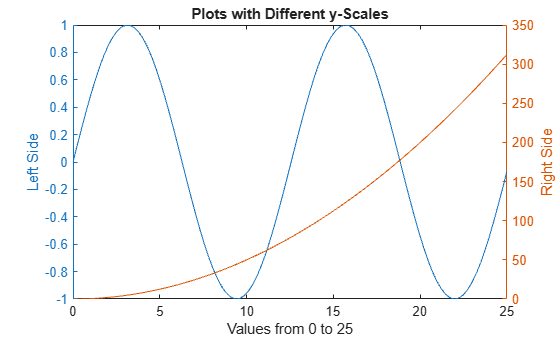### 基于每一侧绘制其他数据图

```hold on yyaxis left y2 = sin(x/3); plot(x,y2); y3 = sin(x/4); plot(x,y3); yyaxis right load count.dat; m = mean(count,2); e = std(count,1,2); errorbar(m,e) hold off```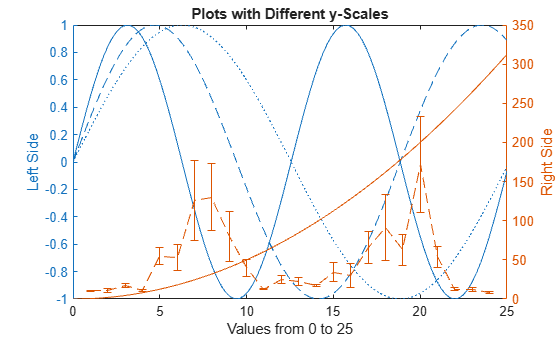### 清除坐标区的一侧

```yyaxis right cla```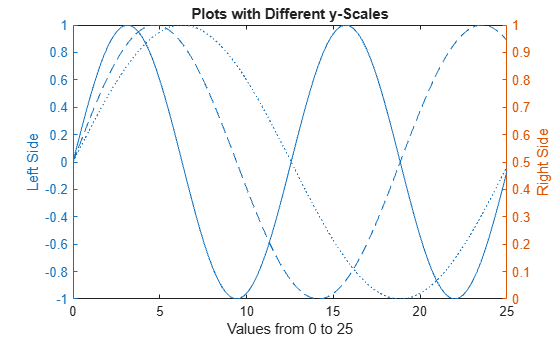### 清除坐标区并删除右侧 y 轴

`cla reset`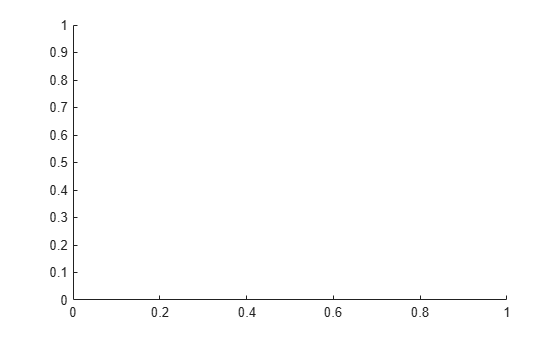```xx = linspace(0,25); yy1 = sin(xx/4); yy2 = sin(xx/5); yy3 = sin(xx/6); plot(xx,yy1,xx,yy2,xx,yy3)```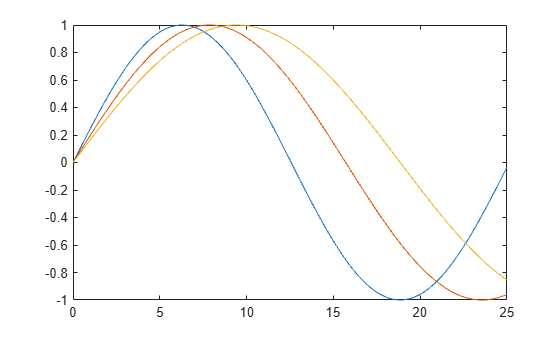### 将第二个 y 轴添加到现有图形

```yyaxis right rr1 = exp(xx/6); rr2 = exp(xx/8); plot(xx,rr1,xx,rr2)```